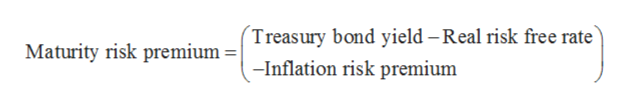A 2-year Treasury security currently earns 1.79 percent. Over the next two years, the real risk-free rate is expected to be 1.35 percent per year and the inflation premium is expected to be 0.35 percent per year. Calculate the maturity risk premium on the 2-year Treasury security. (Round your answer to 2 decimal places.) Maturity risk premium ____.__%

Question

A 2-year Treasury security currently earns 1.79 percent. Over the next two years, the real risk-free rate is expected to be 1.35 percent per year and the inflation premium is expected to be 0.35 percent per year. Calculate the maturity risk premium on the 2-year Treasury security. (Round your answer to 2 decimal places.)

Maturity risk premium ____.__%

Step 1

Maturity risk premium can be defined as the additional benefit an investor can earn on bonds having longer maturity period.

Step 2

The formula to calculate maturity risk premium is given below:help_outlineImage TranscriptioncloseTreasury bond yield - Real risk free rate Maturity risk premium -Inflation risk premium fullscreen
Step 3

Substitute 1.79% for treasury bond yield, 1.35% for real risk-free ra...

Want to see the full answer?

See Solution

Want to see this answer and more?

Our solutions are written by experts, many with advanced degrees, and available 24/7

See Solution
Tagged in